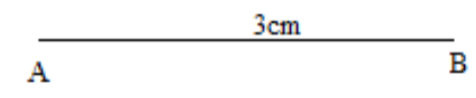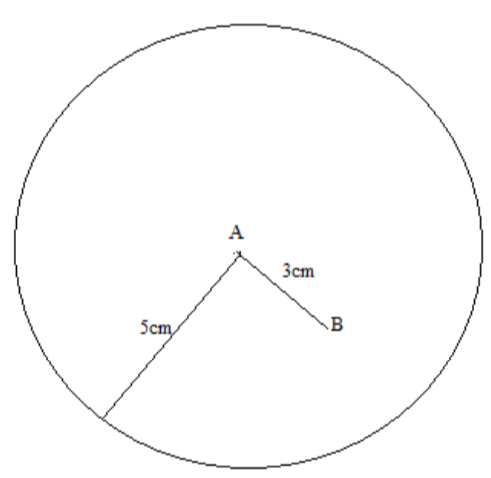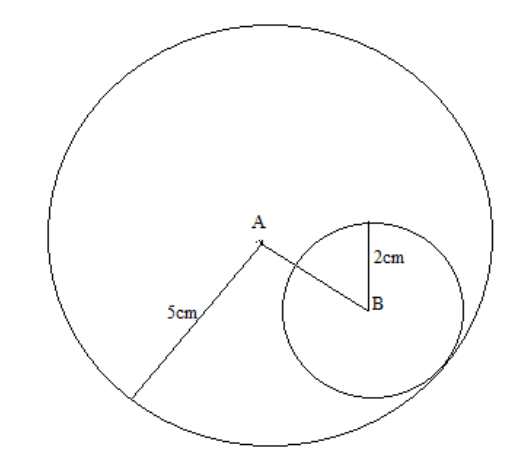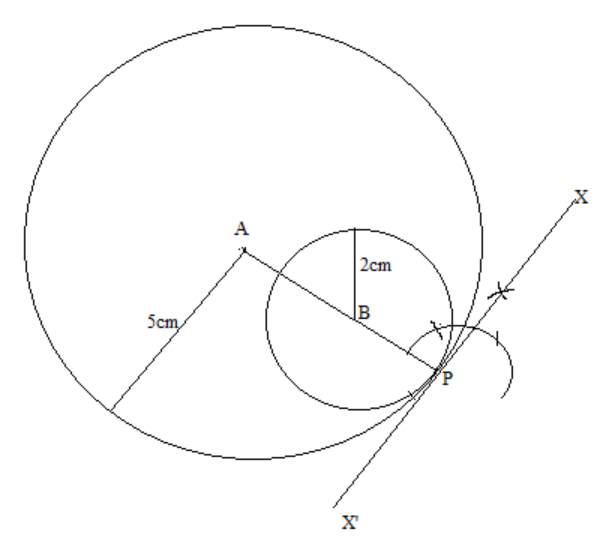QuestionAnswers

# Construct a direct common tangent to two circles of radii 5cm and 2 cm whose centres are 3 cm and 2 cm apart.Hint: Here we will draw the required construction step by step. First we draw a circle of radius 5cm and then taking a point 3cm apart as the centre, we will draw another radius of 2cm. Finally at the point of contact of the two circles, we will have an angle of 90 degrees. The line that forms is the tangent.

The steps for making the required construction are as follow:
Step1: Draw a line segment of 3cmStep2: Then draw a circle of radius 5cm taking as the centre. The circle is drawn using a compass.Step3: Now draw another circle of radius 2cm taking B as the centre. Since the distance between the centres is 3cm. So, both the circles will touch at the point P.Step4: Now to draw the common tangent, we will make an angle of 90 degree at the common point of the two circles. So, we will join AP and then draw a perpendicular to AP at vertex P.
The perpendicular XX’ formed by joining the two points will be the required common tangent of two circles.Note In the construction related to the tangent on the circle, the important point is that you should know that the tangent drawn at a point on a circle makes an angle of 90 degree with radius drawn at that point. Here since both circles are touching internally, so only one common tangent is possible.

To Determine the Mass of Two Different Objects Using a Beam BalanceCBSE Class 10 Maths Chapter 10 - Circles FormulaHow to Make a Model of Seasons?Area of a Sector of a Circle FormulaHow to Find Square Root of a NumberHow To Make A Buzzer?How to Make A VolcanoHow to Make a Wooden Generator?How to Make a Potato Clock?CBSE Class 10 Maths Chapter 8 - Introduction to Trigonometry FormulaImportant Questions for CBSE Class 10 Maths Chapter 12 - Areas Related to CirclesImportant Questions for CBSE Class 6 English A Pact with The Sun Chapter 1 - A Tale of Two BirdsImportant Questions for CBSE Class 10 Maths Chapter 10 - CirclesImportant Questions for CBSE Class 9 Maths Chapter 10 - CirclesImportant Questions for CBSE Class 8 English Honeydew Chapter 7 - A Visit to CambridgeImportant Questions for CBSE Class 10 Maths Chapter 8 - Introduction to TrigonometryImportant Questions for CBSE Class 10 Maths Chapter 3 - Pair of Linear Equations in Two VariablesImportant Questions for CBSE Class 6 English A Pact with The Sun Chapter 10 - A Strange Wrestling MatchImportant Questions for CBSE Class 6 English A Pact with The Sun Chapter 9 - What Happened to The ReptilesImportant Questions for CBSE Class 8 Maths Chapter 13 - Direct and Inverse ProportionsCBSE Class 10 Hindi A Question Paper 2020Hindi A Class 10 CBSE Question Paper 2009Hindi A Class 10 CBSE Question Paper 2015Hindi A Class 10 CBSE Question Paper 2016Hindi A Class 10 CBSE Question Paper 2012Hindi A Class 10 CBSE Question Paper 2010Hindi A Class 10 CBSE Question Paper 2008Hindi A Class 10 CBSE Question Paper 2014Hindi A Class 10 CBSE Question Paper 2007Hindi A Class 10 CBSE Question Paper 2013NCERT Solutions for Class 10 Maths Chapter 12 Areas Related to CirclesRD Sharma Solutions for Class 10 Maths Chapter 15 - Areas Related to CirclesNCERT Solutions for Class 10 Maths Chapter 10 CirclesRD Sharma Solutions for Class 10 Maths Chapter 10 - CirclesNCERT Solutions for Class 9 Maths Chapter 10 CirclesRS Aggarwal Class 10 Solutions - CirclesNCERT Solutions for Class 10 Maths Chapter 12 Areas Related to Circles in HindiNCERT Exemplar for Class 10 Maths Chapter 11 - Areas Related to Circles (Book Solutions)NCERT Solutions for Class 10 Maths Chapter 12 Areas Related to Circles (Ex 12.2) Exercise 12.2NCERT Solutions for Class 10 English First Flight Chapter 1 - A Letter to God